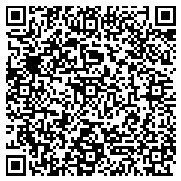### About: Scalar curvature

Permalink

an Entity references as follows:

In Riemannian geometry, the scalar curvature (or the Ricci scalar) is the simplest curvature invariant of a Riemannian manifold. To each point on a Riemannian manifold, it assigns a single real number determined by the intrinsic geometry of the manifold near that point. Specifically, the scalar curvature represents the amount by which the volume of a small geodesic ball in a Riemannian manifold deviates from that of the standard ball in Euclidean space. In two dimensions, the scalar curvature is twice the Gaussian curvature, and completely characterizes the curvature of a surface. In more than two dimensions, however, the curvature of Riemannian manifolds involves more than one functionally independent quantity.Graph IRICount
http://dbpedia.org164 triples
Faceted Search & Find service v1.17_git51

Alternative Linked Data Documents: PivotViewer | iSPARQL | ODE     Raw Data in: CXML | CSV | RDF ( N-Triples N3/Turtle JSON XML ) | OData ( Atom JSON ) | Microdata ( JSON HTML) | JSON-LD    AboutThis work is licensed under a Creative Commons Attribution-Share Alike 3.0 Unported License.
OpenLink Virtuoso version 08.03.3321 as of Jun 2 2021, on Linux (x86_64-generic-linux-glibc25), Single-Server Edition (61 GB total memory)
Copyright © 2009-2021 OpenLink Software